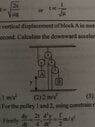# Four pulley system

Gold Member
Homework Statement:
The vertical displacement of block A in meters is ##y=\frac{t^2}4## where t is in sec. Calculate the downward acceleration of block B.
Relevant Equations:
##F=ma##
We can differentiate twice the y displacement with respect to time t and get the acceleration of block B. $$a_B= \frac12 m/s^2$$.
But I don’t think it’s that simple.

#### Attachments

•image.jpg
23 KB · Views: 44

Homework Helper
You think correctlyBut at least you have the acceleration of A.
How is the position of B in relation to A ? (if A moves up 1 m, how much does B move?)

## \ ##

Gold Member
You think correctlyHow is the position of B in relation to A ? (if A moves up 1 m, how much does B move?)
Of course 1 m since all are connected with unstrechable ropes.

Homework Helper
Your logic is faulty. Think again. Number the pulleys 1,2,3,4 left to right. If 1 goes up by 1 unit, 2 stays in position unchanged, 3 ... etc.

##\ ##

Gold Member
Your logic is faulty. Think again. Number the pulleys 1,2,3,4 left to right. If 1 goes up by 1 unit, 2 stays in position unchanged, 3 ... etc.

##\ ##
Oh like that!
1 goes up by 1 unit then 2 stays in place, 3 and 4 goes down by 1 unit.